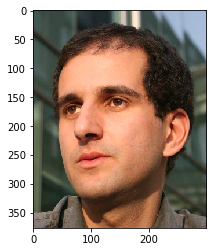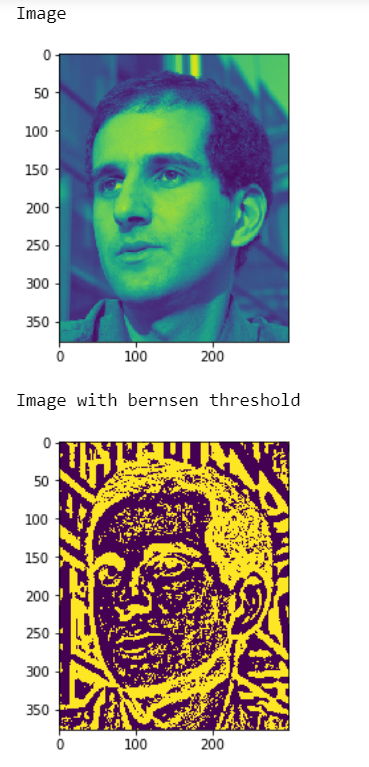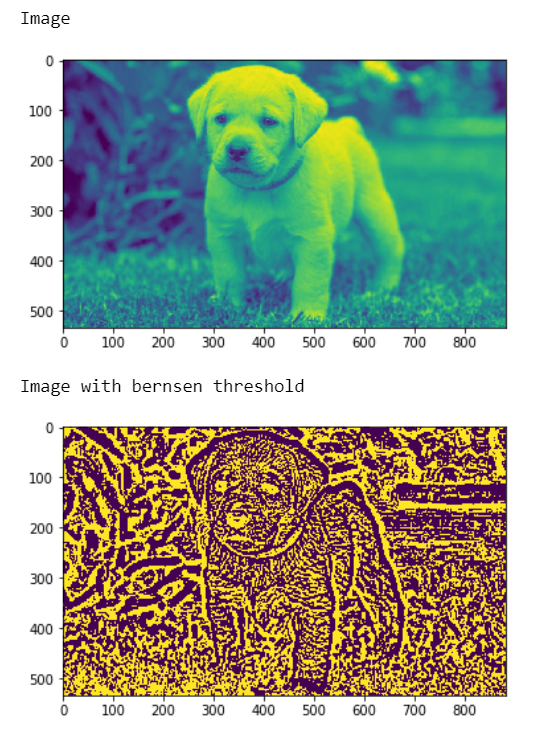# Mahotas – Bernsen local thresholding

In this article we will see how we can implement bernsen local thresholding in mahotas. Bernsen’s method is one of locally adaptive binarization methods developed for image segmentation. In this study, Bernsen’s locally adaptive binarization method is implemented and then tested for different gray scale images.

In this tutorial we will use “luispedro” image, below is the command to load it.

`mahotas.demos.load('luispedro')`

Below is the luispedro imageIn order to do this we will use `mahotas.thresholding.bernsen` method

Syntax : mahotas.thresholding.bernsen(image, constrast_threshold, global_threshold)

Argument : It takes image object and two integer as argument

Return : It returns image object

Note : Input image should be filtered or should be loaded as grey

In order to filter the image we will take the image object which is numpy.ndarray and filter it with the help of indexing, below is the command to do this

`image = image[:, :, 0]`

Example 1:

 `# importing required librries ` `import` `mahotas ` `import` `mahotas.demos ` `import` `numpy as np ` `from` `pylab ``import` `imshow, gray, show ` `from` `os ``import` `path ` ` `  `# loading the image ` `photo ``=` `mahotas.demos.load(``'luispedro'``) ` ` `  ` `  `# loading image as grey ` `photo ``=` `mahotas.demos.load(``'luispedro'``, as_grey ``=` `True``) ` ` `  `# converting image type to unit8 ` `# beacuse as_grey returns floating values ` `photo ``=` `photo.astype(np.uint8) ` ` `  `# showing original image ` `print``(``"Image"``) ` `imshow(photo) ` `show() ` ` `  `# bernsen threshold ` `photo ``=` `mahotas.thresholding.bernsen(photo, ``7``, ``200``) ` ` `  ` `  `print``(``"Image with bernsen threshold"``) ` ` `  `# showing image ` `imshow(photo) ` `show() `

Output :Example 2:

 `# importing required libraries ` `import` `mahotas ` `import` `numpy as np ` `from` `pylab ``import` `imshow, show ` `import` `os ` ` `  ` `  `# loading iamge ` `img ``=` `mahotas.imread(``'dog_image.png'``) ` `     `  ` `  `# setting filter to the image ` `img ``=` `img[:, :, ``0``] ` ` `  `print``(``"Image"``) ` ` `  `# shoing the image ` `imshow(img) ` `show() ` ` `  ` `  `# bernsen threshold ` `img ``=` `mahotas.thresholding.bernsen(img, ``5``, ``100``) ` ` `  ` `  `print``(``"Image with bernsen threshold"``) ` ` `  `# showing image ` `imshow(img) ` `show() `

Output :My Personal Notes arrow_drop_upCheck out this Author's contributed articles.

If you like GeeksforGeeks and would like to contribute, you can also write an article using contribute.geeksforgeeks.org or mail your article to contribute@geeksforgeeks.org. See your article appearing on the GeeksforGeeks main page and help other Geeks.

Please Improve this article if you find anything incorrect by clicking on the "Improve Article" button below.

Article Tags :

Be the First to upvote.

Please write to us at contribute@geeksforgeeks.org to report any issue with the above content.